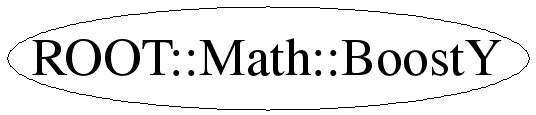# class ROOT::Math::BoostY

```
Class representing a Lorentz Boost along the Y axis, by beta.
For efficiency, gamma is held as well.

@ingroup GenVector

```

## Function Members (Methods)

public:
 ~BoostY() ROOT::Math::BoostY::Scalar Beta() const ROOT::Math::BoostY::XYZVector BetaVector() const ROOT::Math::BoostY BoostY() ROOT::Math::BoostY BoostY(ROOT::Math::BoostY::Scalar beta_y) ROOT::Math::BoostY BoostY(const ROOT::Math::BoostY&) ROOT::Math::BoostY::Scalar Gamma() const void GetComponents(ROOT::Math::BoostY::Scalar& beta_y) const void GetLorentzRotation(ROOT::Math::BoostY::Scalar* r) const ROOT::Math::BoostY Inverse() const void Invert() bool operator!=(const ROOT::Math::BoostY& rhs) const ROOT::Math::LorentzVector > operator()(const ROOT::Math::LorentzVector >& v) const ROOT::Math::LorentzVector > operator*(const ROOT::Math::LorentzVector >& v) const ROOT::Math::BoostY& operator=(const ROOT::Math::BoostY&) bool operator==(const ROOT::Math::BoostY& rhs) const void Rectify() void SetBeta(ROOT::Math::BoostY::Scalar beta) void SetComponents(ROOT::Math::BoostY::Scalar beta_y)

## Data Members

public:
 enum ELorentzRotationMatrixIndex { kLXX kLXY kLXZ kLXT kLYX kLYY kLYZ kLYT kLZX kLZY kLZZ kLZT kLTX kLTY kLTZ kLTT }; enum EBoostMatrixIndex { kXX kXY kXZ kXT kYY kYZ kYT kZZ kZT kTT };
private:
 ROOT::Math::BoostY::Scalar fBeta beta Y of the Boost ROOT::Math::BoostY::Scalar fGamma gamma of the Boost

## Class Charts## Function documentation

BoostY()
``` ========== Constructors and Assignment =====================

Default constructor (identity transformation)

```
explicit BoostY(Scalar beta_y)
```Construct given a Scalar beta_y

```
`{ SetComponents(beta_y); }`
void Rectify()
``` The compiler-generated copy ctor, copy assignment, and dtor are OK.

Re-adjust components to eliminate small deviations from a perfect
orthosyplectic matrix.

```
SetComponents(ROOT::Math::BoostY::Scalar beta_y)
``` ======== Components ==============

Set components from a Scalar beta_y

```
GetComponents(ROOT::Math::BoostY::Scalar& beta_y) const
```Get components into a Scalar beta_y

```
Scalar Beta()
```Retrieve the beta of the Boost

```
`{ return fBeta; }`
Scalar Gamma()
```Retrieve the gamma of the Boost

```
`{ return fGamma; }`
void SetBeta(ROOT::Math::BoostY::Scalar beta)
```Set the given beta of the Boost

```
`{ SetComponents(beta); }`
XYZVector BetaVector()
GetLorentzRotation(ROOT::Math::BoostY::Scalar* r) const
```Get elements of internal 4x4 symmetric representation, into a data
array suitable for direct use as the components of a LorentzRotation
Note -- 16 Scalars will be written into the array; if the array is not
that large, then this will lead to undefined behavior.

```
operator()(const ROOT::Math::LorentzVector<ROOT::Math::PxPyPzE4D<double> >& v) const
``` =========== operations ==============

Lorentz transformation operation on a Minkowski ('Cartesian')
LorentzVector

```
A4Vector operator*(const ROOT::Math::LorentzVector<ROOT::Math::PxPyPzE4D<double> >& v) const
```Overload operator * for rotation on a vector

```
void Invert()
```Invert a BoostY in place

```

```Return inverse of  a rotation

```
return ! operator==(const ROOT::Math::BoostY& rhs) const

Last update: root/mathcore:\$Id: BoostY.h 21503 2007-12-19 17:34:54Z moneta \$
Copyright (c) 2005 ROOT FNAL MathLib Team *

This page has been automatically generated. If you have any comments or suggestions about the page layout send a mail to ROOT support, or contact the developers with any questions or problems regarding ROOT.